# A circle is inscribed in a rhombus | CAT Geometry

## CAT 2020 - Slot 1 - Quantitative Aptitude - Geometry - Question 18 - A circle is inscribed in a rhombus

Q.18: A circle is inscribed in a rhombus with diagonals 12 cm and 16 cm. The ratio of the area of the circle to the area of the rhombus is

##### 1.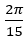2.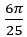3.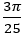4.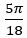Correct Answer: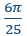Diagonals of a rhombus are perpendicular bisectors of each other

If diagonals are 12 and 16

Half of diagonals are 6 and 8 third side will become 10 = side of rhombus

Radius of the circle will be perpendicular to the side of the rhombus

Because side of the rhombus is tangent to the circle

The triangle is taken by half a diagonal (6) and (8) from the original figure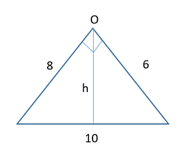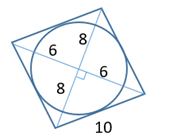Just equating the areas

1/2 × 8 × 6 = 1/2 × 10 × h

h = 24/5 = same as radius of the circle (r)

Now, area of circle : area of rhombus

π × 24/5 × 24/5 : 1/2 × 12 × 16

6π : 25

### Past Year Question Paper & SolutionsCounselling Session
By IIM Mentor

#### Free Material Area